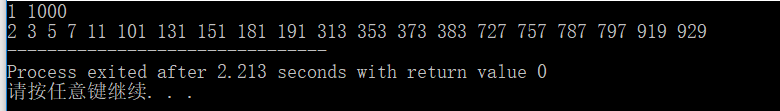# 笨方法写C语言程序：回文素数

1.既然正读反读一样是它的定义，那么，假设输入的数字是number，我利用取余和取整循环着从后向前取出它的每一位，将其按位存入数组，这样就实现了反读。
2.接下来把这些数组里的数字循环着乘上10再加上下一位就可以得出反读后的数sum。
3.判断反读出来的数sum与原数number是否相等。一样的话即为回文数，否则不是。

（我个人觉得回文数更特殊，要少于素数，但是我没有证据。所以我在程序中先判断是不是回文数，在回文数的基础上再判断是不是素数。我认为这样可以提高效率。）

（由于我对循环的逻辑不甚了解所以我采用了计数的方法进行素数判断，详情看代码吧）

#include<stdio.h>
int judgehui(int number)//此函数判断是否为回文数
{
int c={0};
int initial=number;
int i=0;
int num=0;
while(number>0)
{
c[i++]=number%10;//取末位
number=number/10;//舍去末位
}
for(int k=0;k<i;k++)//计算反读的数值
{
num=num*10;
num+=c[k];
}
if(num==initial)
return 1;//一样输出1
else
return 0;
}
int judgesu(int number)//判断是否为素数
{
int count2=0;
int mark=0;
if(number==0||number==1)//0和1是非素数
mark=0;
if(number==2)//2是素数
mark=1;
if(number>2)
{
for(int i=2;i<number;i++)
{
if(number%i!=0)//对每一个不能整除的数都计数
{
count2++;
}
}
if(count2==number-2)//若该数前面除了0，1以外的所有数都与它互质
mark=1;//则为素数
}
return mark;
}
int main()
{
int a,b;
scanf("%d%d",&a,&b);
int number;
for(number=a;number<b;number++)
{
if(judgehui(number)==1)//判断是否为回文数
{
if(judgesu(number)==1)//判断是否为素数
printf("%d ",number);//输出该数
}
}
return 0;
}12-1601-251万+
12-073万+
12-08602
05-042万+
03-19605
04-22136
04-1266
12-107362
09-18
12-031076
12-051666
10-01566
12-19126
09-233万+
11-187337
03-063630